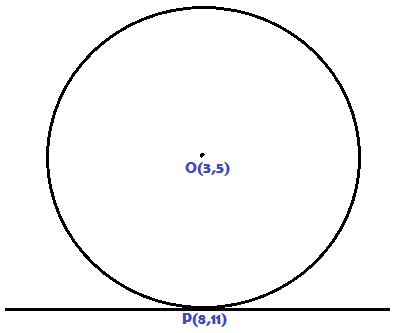# Because $\frac{dy}{dx}$ has killed all the creativity inside!

Geometry Level 3As shown in the figure, a circle centered at $O=(3,5)$ bears a point $P=(8,11)$ hosting a line tangent to the circle. Can you find out the equation of that tangent line without using calculus?

If the equation of the line can be expressed in the form $ax+by+c=0$, where $a$,$b$ and $c$ are integers and $\gcd(a,b,c)=1$, then enter $|abc|$ as your answer.

 Notations:

×